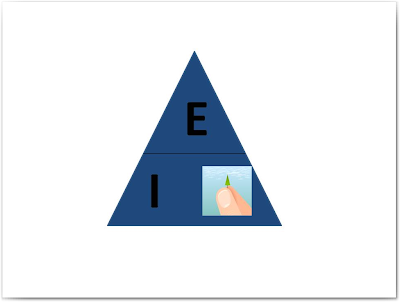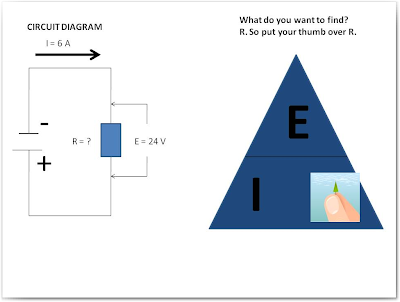## Wednesday, August 26, 2009

### The Basic Concept of Ohm's Law

I just can't wait for another morning without my new post here in Learn Electrical Engineering for Beginners. It's going interesting here because I've noticed that there are new another batch of subscriber who want to learn about Electrical Engineering. Since you've found this site, you're halfway to learning the Electrical Engineering. For those who are new in this site, you can still catch up with our previous 2 last topics here.
Ok, let's begin with our new basic topic.

The Relationship of Voltage, Current, and Resistance

This next topic, you will learn about Ohm's Law, this is one of the most basic and important that you will use throughout your career here in Electrical Engineering.

The concept is just simple: given a constant resistance in a circuit, the current flow increases as the voltage applied to the circuit increases. Given a constant voltage (emf) applied to the circuit, current flow decreases as the resistance of the circuit increases. You can finalize these ideas or concepts as follows: Current flow in the circuit increases as the voltage is increased, and decreases as the resistance is increased.

The relationship of voltage, current, and resistance was studied by German Physicist, George Simon Ohm. His statement of this relationship, called Ohm's Law. Obviously, the title of the law was derived itself on his name. This is one of the fundamental laws of Physics.

What does this vital laws state? This is simple as:

With Constant Resistance
• lower voltage gives small current.
• higher voltage gives large current.
With Constant EMF
• lower resistance passes large current.
• higher resistance passes small current.
In a more technical expression, you can state it as:

Ohm's Law states that the current flowing in a circuit is directly proportional to the voltage applied (emf) and inversely proportional to the resistance.

It is also possible to express Ohm's Law as a mathematical equation (relationship) as further indicated below:

CURRENT = EMF / RESISTANCE

In electrical terms (notation), current is always represented by the letter "I", resistance by the letter "R" and the voltage by the letter "E" or you can used letter "V". You can therefore rewrite the mathematical given above in another way like what as shown below:

I = E / R

Using our simple algebra, you can also derived it in these ways:

E = I x R or as R = E / I

Which of the three ways ( formulas) of expressing Ohm's Law you might choose to employ depends on two things: 1. what facts you know and 2. what facts you need to know about the circuit you are considering.

Ohhh... I think you've got a headache on how to remember those formulas above. Let's have a little trick on how you can remember it better. Let's draw a triangle with a horizontal line across it half-way up from its base. Write letter E in the small triangle, which has been formed above the line, and the letters I and R below the line, it will look like as what as shown below. This is what you called the magic triangle!Ok! now consider a circuit in which you know the values of any two of the three factors - voltage, current, and resistance- and want to find out the third. The rule for working the magic triangle to give the correct formula is as follows:

Put your thumb over the letter in the triangle whose value you want to know- and the formula for calculating that value is given by the two remaining letters.
Here is how to do it:

1. If you know the values of current and resistance in the circuit. But the value of voltage is unknown and you cannot measure it because of some reason ( no available voltmeter to use). Draw the magic triangle, put your thumb you want to calculate, which in this case the E- and you are left with the formula you need. ---- I X R.2. You know the values of current and voltage, but in this case you have no ohmmeter to measure the resistance. Put your thumb over the letter R and you are left with the formula E / I. Substitute the known values for E and I, and your answer is R.3. The voltage and the resistance of a circuit are known to you; but in this case the ammeter you need to measure the current is lost or broken. Put your thumb over the symbol I. and read off the formula you need: E/R.Ohm's law cannot work properly if you will not know on how to expressed its values in the correct units of measurement. The next topic will show you the Ohm's Law Rules.

Ohm's Law Rules

Ohm's Law will work for you and give you the correct answer in any problem situations which you may try to solve. In the Ohm's Law equation, the first rule is that:

CURRENT is ALWAYS expressed in AMPERES.
VOLTAGE is ALWAYS expressed in VOLTS.
RESISTANCE is ALWAYS expressed in OHMS.

Let's have an example of the above rule.

Take you have a circuit in which you have to measure the resistance to be 100 ohms, and the current to be 300 milliamperes (mA). Obviously, if you use the Ohm's Law without knowing the correct rule mentioned above, you will arrived like this: E = I X R = 100 X 300 = 30,000 your answer will be definitely wrong by a factor of 1,000.

You have to use the conversion tables as what you have learned in Physics, and you must rewrite all factors in the simple expression above in amperes, volts and ohms. When you did this, you will obtain:

E = I X R = 100 X 0.3 = 30 volts - answer

Thus, giving you the correct answer.

There is a second rule in which you must apply whenever you are attempting to solve an Ohm's Law problem involving quantities and values in an electric circuit. The rule is: Always sketch a rough diagram of the circuit you are considering, before you start making calculations based on the values in the circuit which are already known to you. This rule is very useful especially when we already dealing with the more complex circuits.

Let's have an example of the above second rule:

Supposed you have an unknown resistor connected across the battery, and you find by measurement that the voltage across it is 24 volts. You measure the current flowing as 6 amperes. You want to know the resistance of the resistor; but you have no ammeter.

This is how you will solve it.

First, draw the circuit diagram, and fill it in with the information you already have. Sketch out the magic triangle ( illustration given below) . The magic triangle tells you that R = E/ I.

Into this equation, you may substitute the known values and get R = 24 / 6 = 4 ohms - answerSince you know now already the basic concept of Ohm's Law, you are now ready to face the challenges here in Electrical Engineering. On my next post let's discuss some applications about it.

Hope you learn something new basic technique here in Learn Electrical Engineering for Beginners.

Cheers to all and Good night!